# Exploring Data with Scatter Plots by Group in R

rtip
viz
Author

Steven P. Sanderson II, MPH

Published

September 19, 2023

# Introduction

Data visualization is a powerful tool for gaining insights from your data. Scatter plots, in particular, are excellent for visualizing relationships between two continuous variables. But what if you want to compare multiple groups within your data? In this blog post, we’ll explore how to create engaging scatter plots by group in R. We’ll walk through the process step by step, providing several examples and explaining the code blocks in simple terms. So, whether you’re a data scientist, analyst, or just curious about R, let’s dive in and discover how to make your data come to life!

# Prerequisites:

Before we get started, make sure you have R and RStudio installed on your computer. If you haven’t already, you can download them from the official websites: R and RStudio.

# Data Preparation:

For this tutorial, we’ll use a sample dataset called `iris`. It’s included in R and contains information about three different species of iris flowers. To begin, load the dataset:

``````# Load the iris dataset
data(iris)``````

Now, let’s examine the first few rows of the dataset using the `head()` function:

``````# View the first 6 rows of the dataset
``````  Sepal.Length Sepal.Width Petal.Length Petal.Width Species
1          5.1         3.5          1.4         0.2  setosa
2          4.9         3.0          1.4         0.2  setosa
3          4.7         3.2          1.3         0.2  setosa
4          4.6         3.1          1.5         0.2  setosa
5          5.0         3.6          1.4         0.2  setosa
6          5.4         3.9          1.7         0.4  setosa``````

This dataset has four numeric variables: Sepal.Length, Sepal.Width, Petal.Length, and Petal.Width. The fifth variable, Species, represents the different iris species (Setosa, Versicolor, and Virginica). We’ll use this categorical variable to group our data for scatter plots.

# Examples

## Using `ggplot2`

### Creating Scatter Plots by Group:

To create scatter plots by group, we’ll use the popular R package, ggplot2. If you haven’t installed it yet, you can do so using the following command:

``if(!require(ggplot2)){install.packages("ggplot2")}``

Now, let’s load the ggplot2 library:

``````# Load the ggplot2 library
library(ggplot2)``````

#### Example 1: Basic Scatter Plot

Let’s start with a basic scatter plot that shows the relationship between Sepal.Length and Sepal.Width for all iris species. We’ll color the points by species to distinguish them:

``````# Create a basic scatter plot
ggplot(
data = iris,
aes(x = Sepal.Length, y = Sepal.Width, color = Species)) +
geom_point() +
labs(title = "Sepal Length vs. Sepal Width by Species",
x = "Sepal Length",
y = "Sepal Width") +
theme_minimal()``````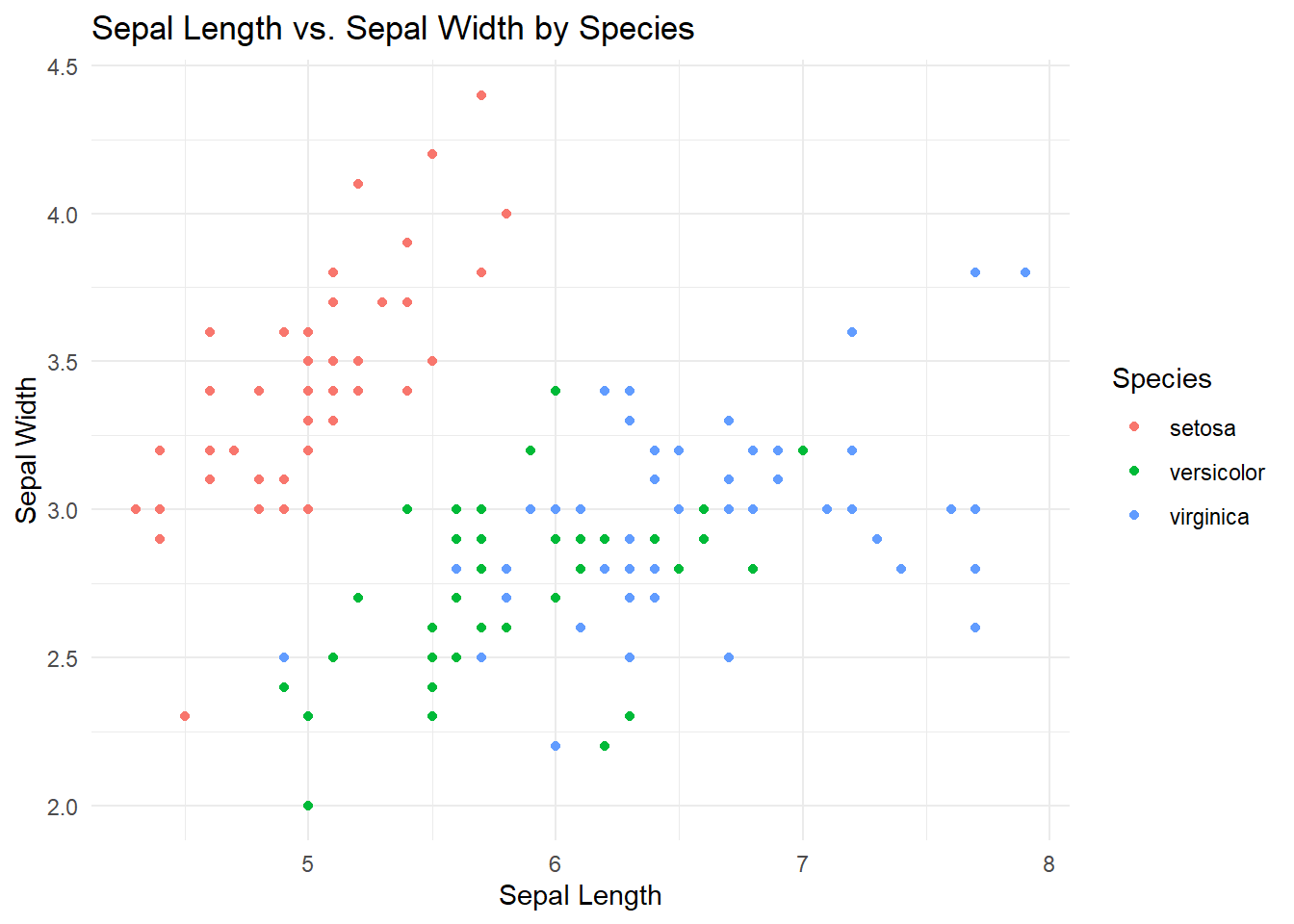In this code: - We specify the dataset (`iris`) and the variables we want to plot. - `geom_point()` adds the points to the plot. - `labs()` is used to add a title and label the axes.

#### Example 2: Faceted Scatter Plot

Now, let’s take it a step further and create separate scatter plots for each iris species using faceting:

``````# Create faceted scatter plots
ggplot(
data = iris,
aes(x = Sepal.Length, y = Sepal.Width, color = Species)) +
geom_point() +
facet_wrap(~Species) +
labs(title = "Sepal Length vs. Sepal Width by Species",
x = "Sepal Length",
y = "Sepal Width") +
theme_minimal()``````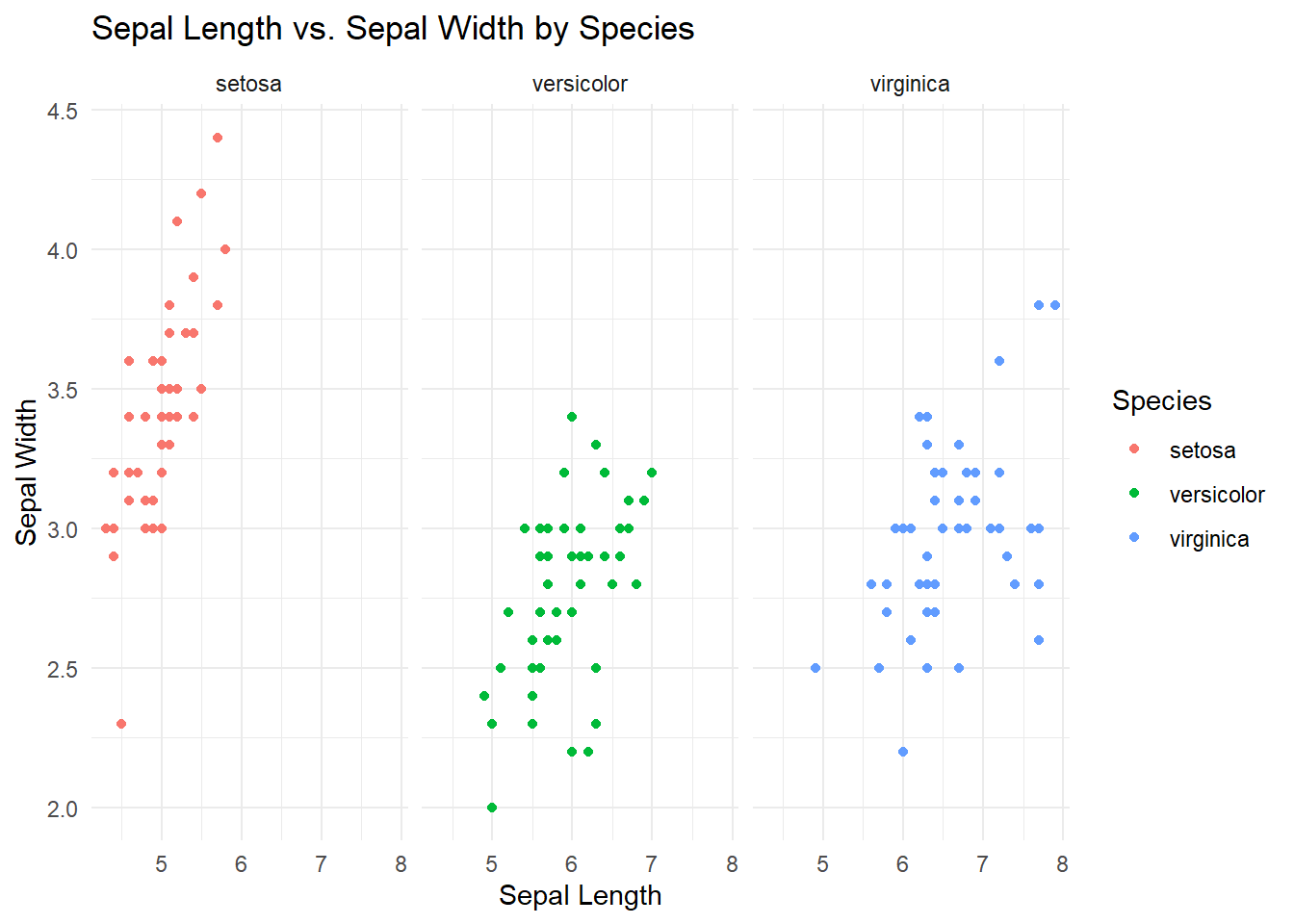In this example, `facet_wrap(~Species)` creates three individual scatter plots, one for each iris species. This makes it easier to compare the species’ characteristics.

#### Example 3: Customized Scatter Plot

Let’s customize our scatter plot further by adding regression lines and adjusting point aesthetics:

``````# Create a customized scatter plot
ggplot(
data = iris,
aes(x = Sepal.Length, y = Sepal.Width, color = Species)) +
geom_point(size = 3, alpha = 0.7, shape = 19) +
geom_smooth(method = "lm", se = FALSE) +
labs(title = "Customized Sepal Length vs. Sepal Width by Species",
x = "Sepal Length",
y = "Sepal Width") +
theme_minimal()``````
```geom_smooth()` using formula = 'y ~ x'``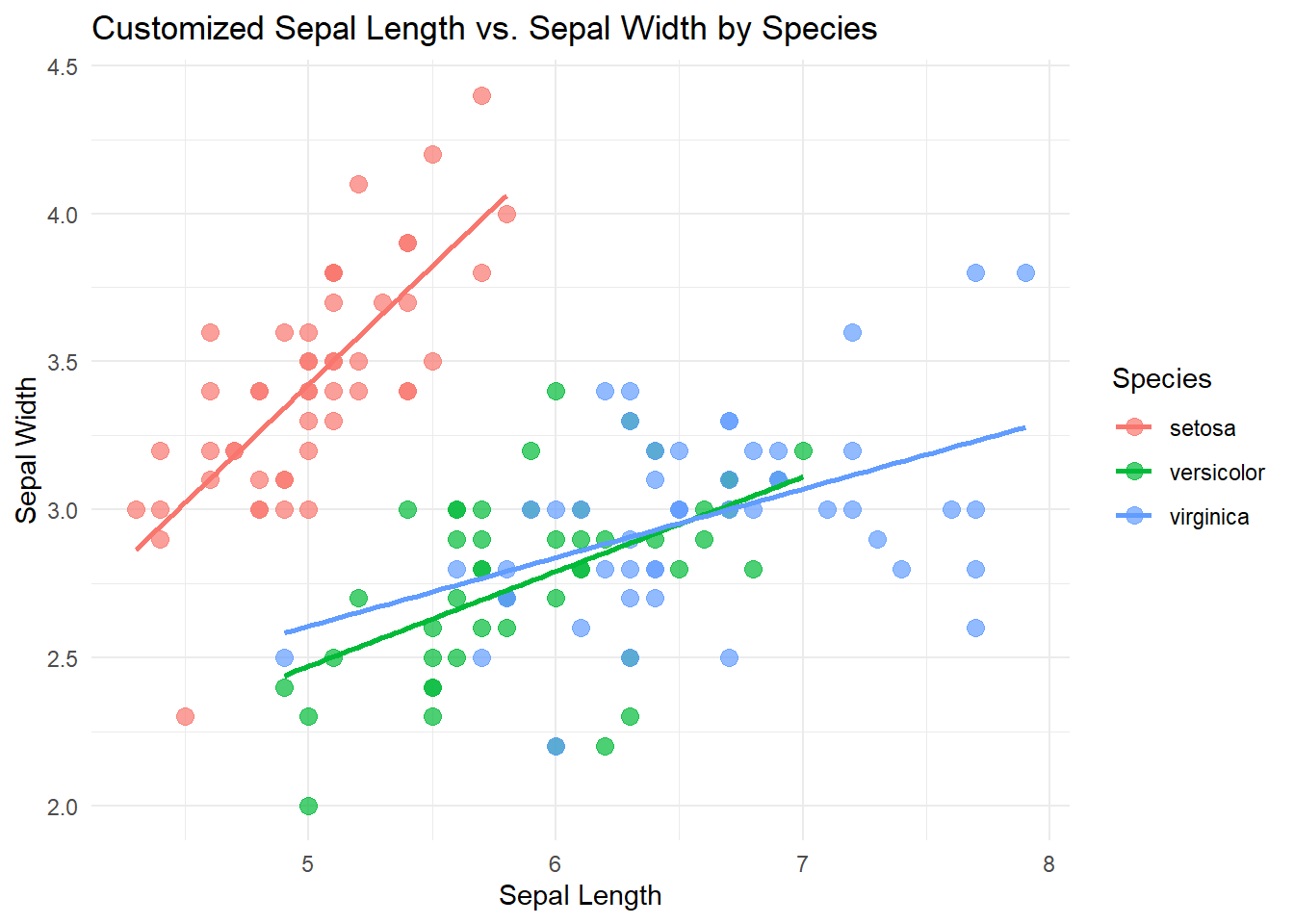In this example: - `geom_point()` now includes size, alpha (transparency), and shape aesthetics. - `geom_smooth()` adds linear regression lines to each group.

## Using Base R

### Example 1: Basic Scatter Plot in Base R

To create a basic scatter plot in base R, we can use the `plot()` function. Here’s how to create a scatter plot of Sepal.Length vs. Sepal.Width by grouping on the “Species” variable:

``````# Create a basic scatter plot
plot(iris\$Sepal.Length, iris\$Sepal.Width, col = iris\$Species,
pch = 19, main = "Sepal Length vs. Sepal Width by Species",
xlab = "Sepal Length", ylab = "Sepal Width")
legend("topright", legend = levels(iris\$Species), col = 1:3, pch = 19)``````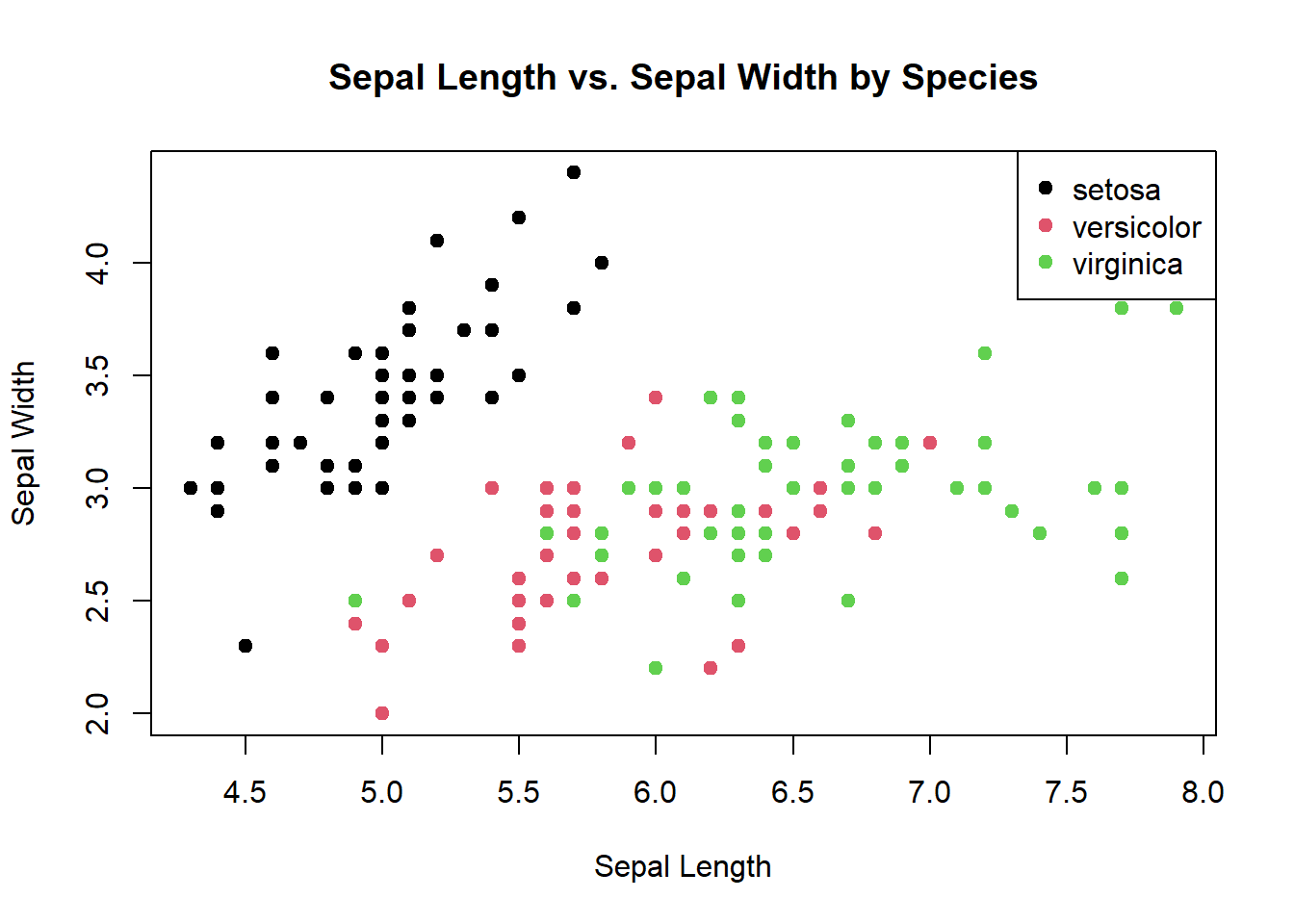In this code: - `plot()` is used to create the scatter plot. - We specify the x and y variables, and we use the `col` argument to color the points by species. - `pch` specifies the point character (shape). - `main`, `xlab`, and `ylab` are used to add a title and label the axes. - `legend()` adds a legend to distinguish the species colors.

### Example 2: Faceted Scatter Plot in Base R

To create faceted scatter plots in base R, we can use the `split()` function to split the data by the “Species” variable and then create individual scatter plots for each group:

``````# Split the data by species
split_data <- split(iris, iris\$Species)

# Create faceted scatter plots
par(mfrow = c(1, 3))  # Arrange plots in one row and three columns
for (i in 1:3) {
plot(split_data[[i]]\$Sepal.Length, split_data[[i]]\$Sepal.Width,
pch = 19, main = levels(iris\$Species)[i],
xlab = "Sepal Length", ylab = "Sepal Width")
}``````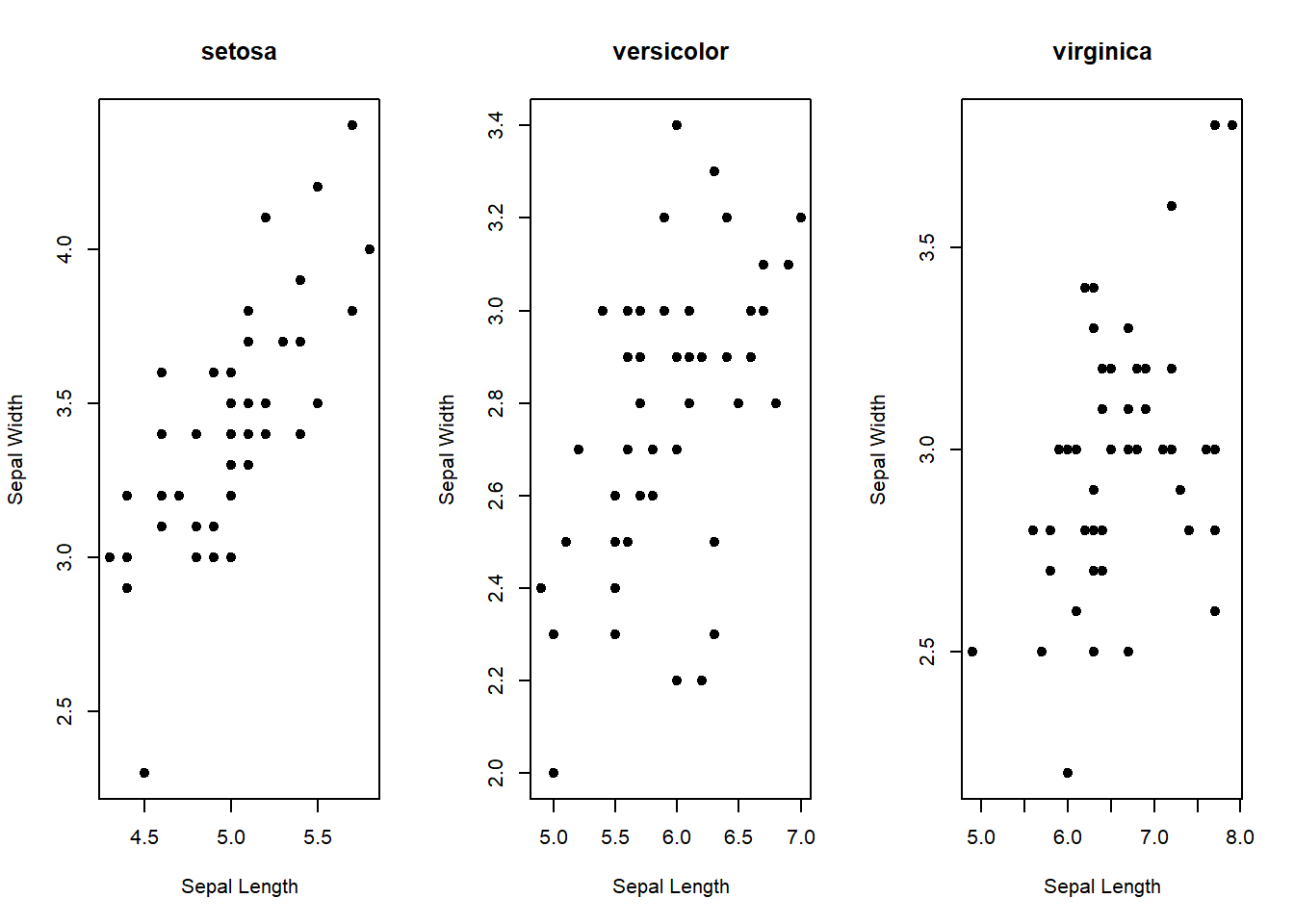``par(mfrow = c(1, 1))``

In this code: - We first use `split()` to split the data into three groups based on the “Species” variable. - Then, we use a `for` loop to create individual scatter plots for each group. - `par(mfrow = c(1, 3))` arranges the plots in one row and three columns.

### Example 3: Customized Scatter Plot in Base R

To create a customized scatter plot in base R, we can adjust various graphical parameters. Here’s an example with customized aesthetics and regression lines:

``````# Create a customized scatter plot with regression lines
plot(iris\$Sepal.Length, iris\$Sepal.Width, col = iris\$Species,
pch = 19, main = "Customized Sepal Length vs. Sepal Width by Species",
xlab = "Sepal Length", ylab = "Sepal Width")
legend("topright", legend = levels(iris\$Species), col = 1:3, pch = 19)

for (i in 1:3) {
group_data <- split_data[[i]]
lm_fit <- lm(Sepal.Width ~ Sepal.Length, data = group_data)
abline(lm_fit, col = i)
}``````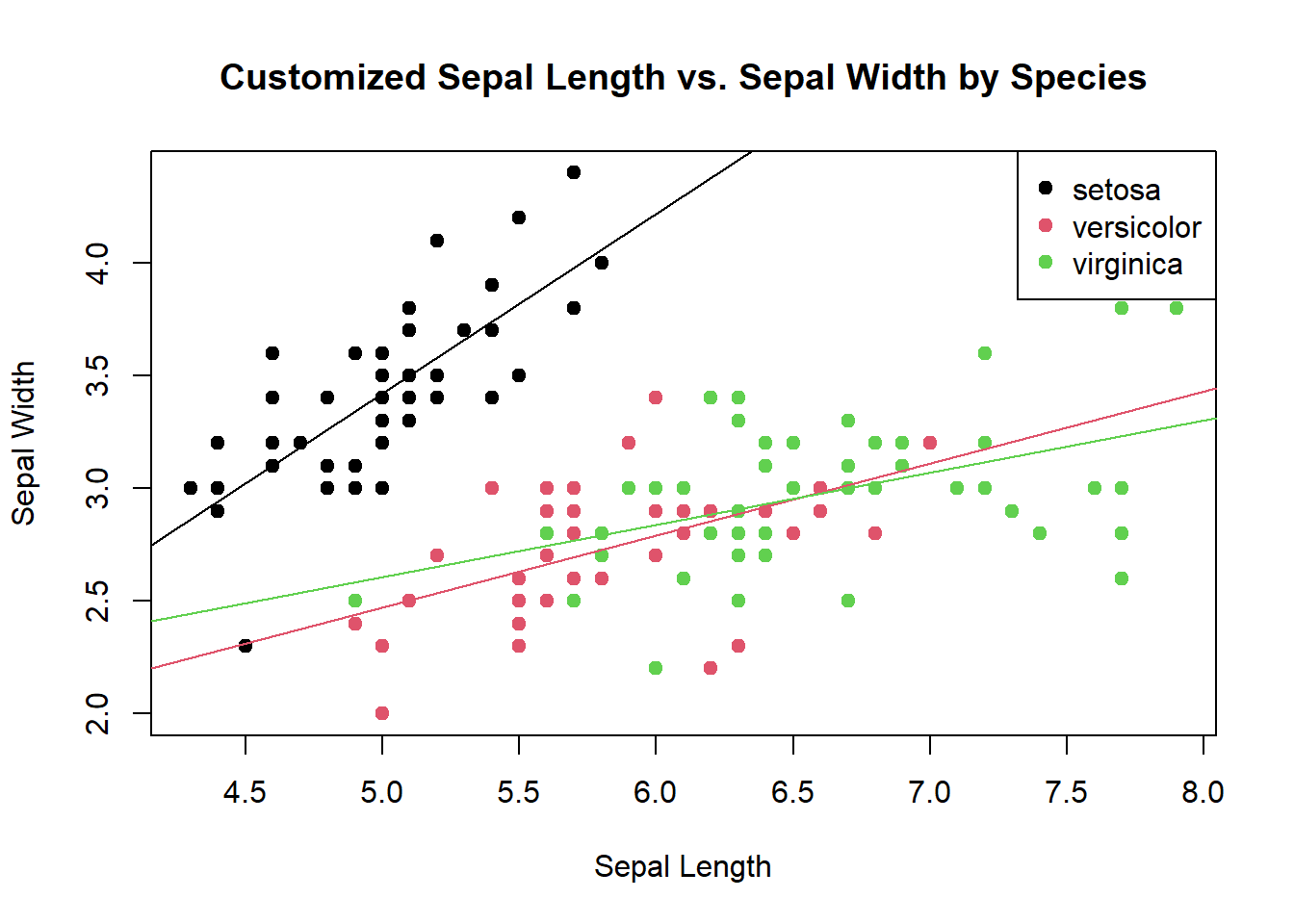In this code: - We add regression lines to each group using a `for` loop and the `abline()` function. - The `lm()` function is used to fit linear regression models to each group separately.

Now you have recreated the scatter plots by group using base R. Feel free to explore more customization options and adapt these examples to your specific needs. Happy coding!

# Conclusion:

Creating scatter plots by group in R allows you to uncover hidden patterns and trends within your data. We’ve explored basic scatter plots, faceted plots, and even customized visualizations. Remember, the power of R lies in its flexibility, so don’t hesitate to experiment and make these examples your own. Try different datasets and variables, change colors, and explore various plotting options to truly harness the power of data visualization in R. Happy coding!